# Blogs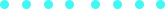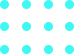### Look Up The A-Stars, Look How They Shine For You

Published on Feb 28, 2018 by onContinuing the series about crowd simulation that I started in this post, today I’ll talk about the A* (A-Star) algorithm. The A* can be thought as a global path planner in your game, where given a set of waypoints in the environment, it will tell to an agent what specific waypoints it has to pass through in order to reach its final goal location. The interactions while moving between waypoints have to be handled by a local planner, but this is subject for other posts.

## A-Star

### Prepare stuff

Let’s start then. First, we need waypoints in our environment. You can place waypoints yourself or place them randomly or use some algorithm to place them in some smarter way. So, for the following scene, the spheres are representing the location of waypoints that I placed arbitrarily.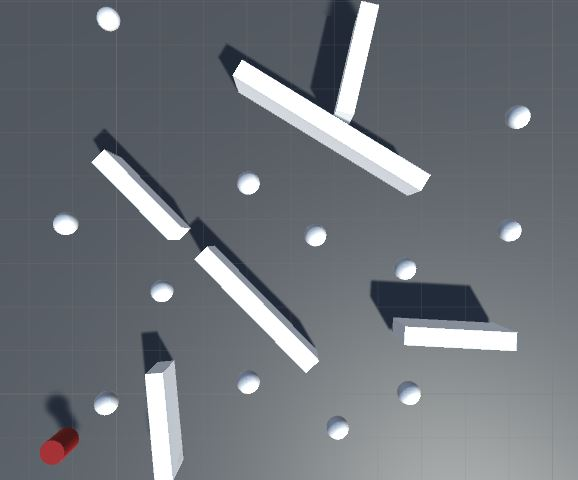### Preprocess stuff

Now, for each pair of different waypoints, we:

• Check if they are neighbors, i.e., there is no obstacle between them. If so, we keep this information.
• Compute the euclidian distance (straight line) between them (even if they are not neighbors).

### Compute path

This is the main part of the algorithm. Here, given a starting waypoint and an ending waypoint, the algorithm has to return a list of waypoints forming the shortest path (minimal cost according to constraints provided) from start to end.

From the starting point, we compute the cost of moving to the neighbors and keep those neighbors as candidates for the next move. We then select the candidate with lesser cost, compute the cost of moving to its non-visited neighbors and add them to the list of candidates, and so on until there are no more candidates to evaluate. Every time we update the cost of moving to a neighbor we keep track of the waypoint where we should move from. This, as it is, is the famous Dijkstra’s algorithm, the difference from the A* will be the addition of a heuristic when sampling the next waypoint candidate.

Here, I will compute the cost of moving from a waypoint A to a waypoint B as `cost(A) + distance(A, B)` where `cost(A)` returns the cost from the starting waypoint to waypoint A and `distance()` returns the euclidian distance between two waypoints. However, in my example, when sampling a candidate I want to get one that has `min(cost(candidate) + distance(candidate, endpoint))`, i.e., I will give preference to the one that has low cost and is close to the ending waypoint at same time. The heuristic allows us to obtain our result more quickly and it can be whatever you want or makes sense in your path finding, not necessarily distances.

The algorithm is something like:

1. Compute the cost of the starting waypoint, which is… 0. Actually, this first value does not matter.
2. Add this waypoint to a list sorted by cost (or priority queue or something with the same purpose).
3. If the list is not empty, take the first waypoint (or last, it depends on how you sort, take the one with lesser cost). Otherwise, go to 7.
4. If the current waypoint is the end waypoint, go to 7.
5. For each neighbor, compute the cost of moving there from the current waypoint. If that cost is lesser then the current cost, set the new cost and set the current waypoint as neighbor’s parent and add this neighbor to the list (adding the distance to the ending point to its cost).
6. Go to 3.
7. If the last waypoint taken is the end waypoint, track back the path using the waypoints’ parent information. Otherwise, there is no path from start to end.

It might be a bit confuse, I didn’t give my best to write that. The following gifs show the difference between A* (left) and Dijkstra (right), where the blue sphere is the ending waypoint, black is a visited waypoint, the range from yellow to red shows the cost (yellow -> low, red -> high) and green is the final path.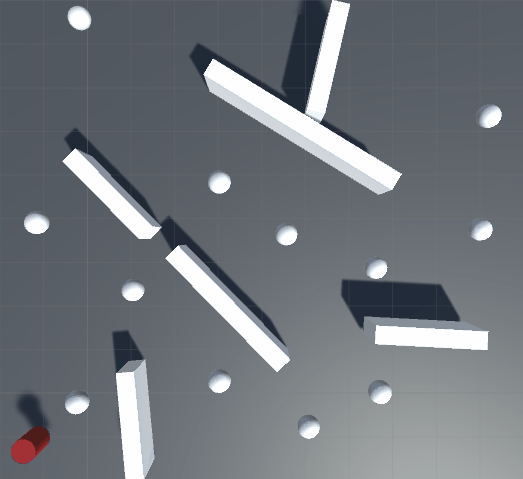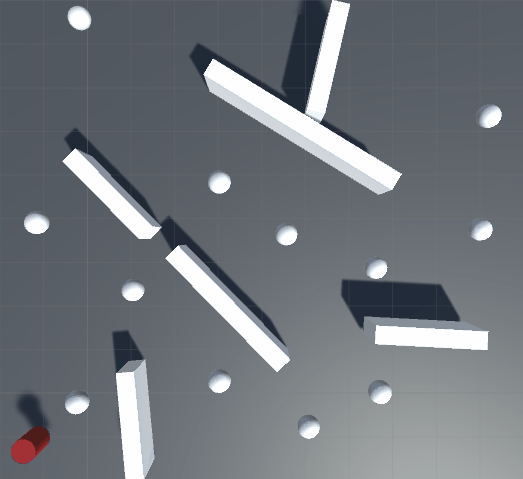### Code

This code shows the preproces and path finding phases. You will find the full source code in the repository (link is in the end of this post). The code will probably change when I start adding more stuff in the repository, but I will try to keep this updated.

``````/// Check for viable paths and compute distances between waypoints.
void Preprocess()
{
if(m_Waypoints != null)
{
_Distances = new float[m_Waypoints.Length,m_Waypoints.Length];

// Clear all list of neighbors.
for(int waypoint1Id = 0; waypoint1Id < m_Waypoints.Length; ++waypoint1Id)
{
Waypoint waypoint1 = m_Waypoints[waypoint1Id];
waypoint1.m_Neighbors.Clear();
waypoint1.id = waypoint1Id;
}

for(int waypoint1Id = 0; waypoint1Id < m_Waypoints.Length; ++waypoint1Id)
{
Waypoint waypoint1 = m_Waypoints[waypoint1Id];
for(int waypoint2Id = waypoint1Id + 1; waypoint2Id < m_Waypoints.Length; ++waypoint2Id)
{
if(waypoint1Id != waypoint2Id)
{
Waypoint waypoint2 = m_Waypoints[waypoint2Id];
Vector3 direction = waypoint2.transform.position - waypoint1.transform.position;
float distance = direction.magnitude;

// If there is no obstacle between them, add each other to own neighborhood.
if(!Physics.Raycast(waypoint1.transform.position,direction.normalized, distance))
{
}

// Store distances.
_Distances[waypoint1Id, waypoint2Id] = distance;
_Distances[waypoint2Id, waypoint1Id] = distance;
}
}
}
}
}

// Store visited waypoints. Once the path is computed clear only the visited ones.
Queue<Waypoint> _VisitedWaypoints = new Queue<Waypoint>();
// list with waypoints and the cost of moving to it, sorted by cost.
SortedList<float, Waypoint> _SortedList = new SortedList<float, Waypoint>();

///
/// Compute a path from startWaypoint to endWaypoint using A-Star algorithm.
///
public Stack<Waypoint> GetPath(Waypoint startWaypoint, Waypoint endWaypoint)
{
Stack<Waypoint> path = new Stack<Waypoint>();

// If endWaypoint has a direct access from startWaypoint, nothing to do here.
if(startWaypoint.IsNeighbor(endWaypoint))
{
path.Push(endWaypoint);
path.Push(startWaypoint);
} else
{
_SortedList.Clear();

// Add startWaypoint with cost of 0.
startWaypoint.m_Cost = 0;
_VisitedWaypoints.Enqueue(startWaypoint);

Waypoint currentWaypoint = null;

// continue while there are waypoints to visit AND the currentWaypoint is not the endWaypoint
while(_SortedList.Count > 0 && (currentWaypoint = _SortedList[_SortedList.Keys]) != endWaypoint)
{
// Mark waypoint as visited and remove from list.
currentWaypoint.m_Visited = true;
_SortedList.RemoveAt(0);

for(int neighborId = 0; neighborId < currentWaypoint.m_Neighbors.Count; ++neighborId)
{
Waypoint neighborWaypoint = currentWaypoint.m_Neighbors[neighborId];

// Check not visited neighbors.
if(!neighborWaypoint.m_Visited)
{
// Is it cheaper to move from the current waypoint to this neighbor or the previous movement to this neighbor was better?
// ==
// The currentWaypoint cost + distance from currentWaypoint to this neighborWaypoint
// is lesser than the current cost of the neighborWaypoint?
float cost = currentWaypoint.m_Cost + _Distances[currentWaypoint.id, neighborWaypoint.id];
if(cost < neighborWaypoint.m_Cost)
{
// if so, keep the new value and say that is better move from currentWaypoint to neighborWaypoint instead.
neighborWaypoint.m_Cost = cost;
neighborWaypoint.m_Previous = currentWaypoint;

// Add neighborWaypoint to the list to be visited, but add to its cost the distance from it to the endWaypoint.
// This is the heuristic we are using for the A-star. This way, the closest ones to the end will be selected first.
_VisitedWaypoints.Enqueue(neighborWaypoint);
}
}
}
}

// No path found.
if(currentWaypoint != endWaypoint)
{
return null;
}

// Track back the path, from end to start, and store in a stack (LIFO).
Waypoint waypoint = endWaypoint;
while(waypoint != null)
{
path.Push(waypoint);
waypoint = waypoint.m_Previous;
}

// Reset settings of visited points.
while(_VisitedWaypoints.Count > 0)
{
_VisitedWaypoints.Dequeue().Reset();
}
}

return path;
}
``````

## Considerations

This is just a naive implementation to give a general idea about the A*. This is an old algorithm and several improvements have been made to it since its first appearance. Also, it would be impossible to describe a general solution, given that each game has its own demands and restrictions. The heuristics used has to be adapted accordingly. For example, one might add cost for slopes in terrain or for areas considered dangerous in the environment.

I created this new separate repository only for crowd simulation stuff. This A* implementation is already there.

The next post in this series will probably be about navigation meshes. Stay tuned.## Similar Stories#### From Built-in to URP

Unity’s Scriptable Render Pipeline represents a great advance on the way that unity deals with graphics, giving more power to the users to customize the pipeline the way they want....#### Look up! The Cook-Torrance!

Continuing the posts of stuff that I should have posted last year but for some reason didn’t. Here, some (not deep) thoughts on Cook-Torrance and lookup textures.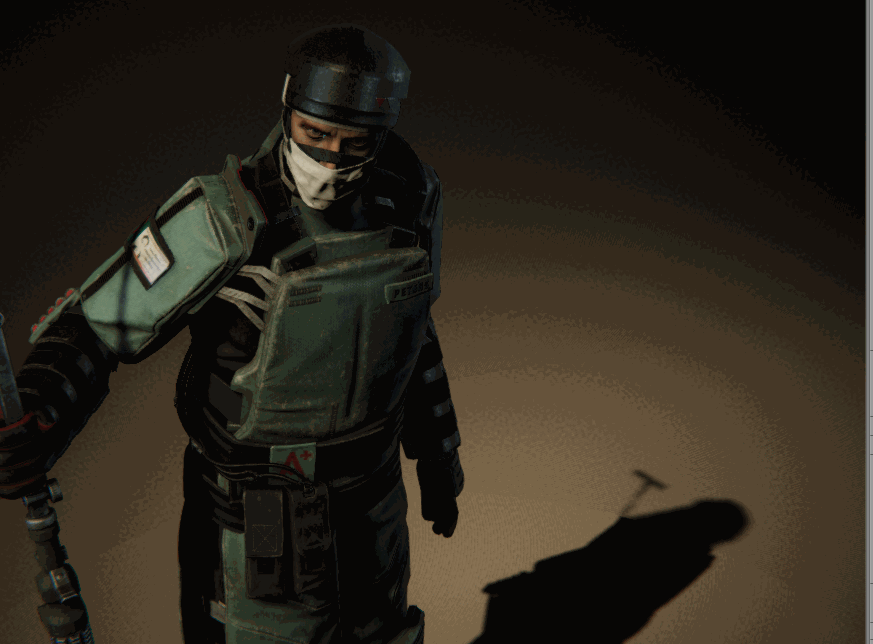#### Halfexperiments with Halftone

Some experiments I did last year with halftone.